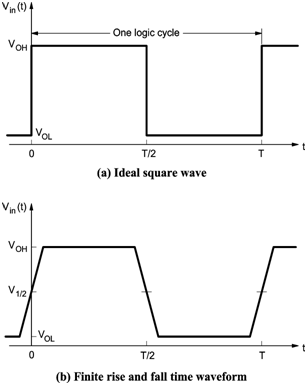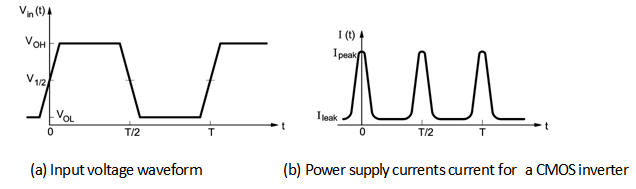Home > Digital CMOS Design > CMOS Inverter > Power Delay Product in CMOS

Power- Delay Product in CMOS

Power- Delay Product in CMOS :

The power-delay product (PDP) is defined as a product of power dissipation and the propagation delay. Figure below shows the shows the PDP input signal waveform.Fig1-Power-Delay-Product-in-CMOS

PDP = Pav tp

The PDP presents a measure of energy, as is apparent from the units (Wsec = Joule).

Assuming that the gate is switched at its maximum possible rate of fmax = 1 /2 tp and ignoring the contributions of the static and direct path currents to the power consumption, we find

PDP = CLV2DD fmax tp = CLV2DD2

The PDP indicates the average energy consumed per switching event (this is, for a

0  1, or 1  0 transition). The power delay product (PDP) is mainly used to determine the performance of a digital integrated circuit.

In order to understand the meaning of the PDP, consider square wave Vin(t)

is applied to the CMOS Inverter; the output voltage Vout(t) then can be drawn in the Figure.The average propagation delay is,

tPHL  D = Ron Cout

tPLH  L = RL Cout

tP  1/2 (Ron + RL)Cout average propagation delay

Current flows only during a switching event so the average current in a logic cycle T can be written as

Iav = 1T [IDn LHtLH + IDn , HLtHL]

In this equation

IDn ,LH  1tLH tLH0 IDn(t)dt.

gives the average current during the rise time while

IDn,HL  1tHL tLH0 IDn(t)dt

is the average fall time current. For a completely symmetric CMOS inverter

IDn,LH = IDHL = IDn,av, So the power-delay product is given by

PDOCMOS = IDn,av VDDtp ffmaxFig2-Power-Delay-Product-in-CMOS